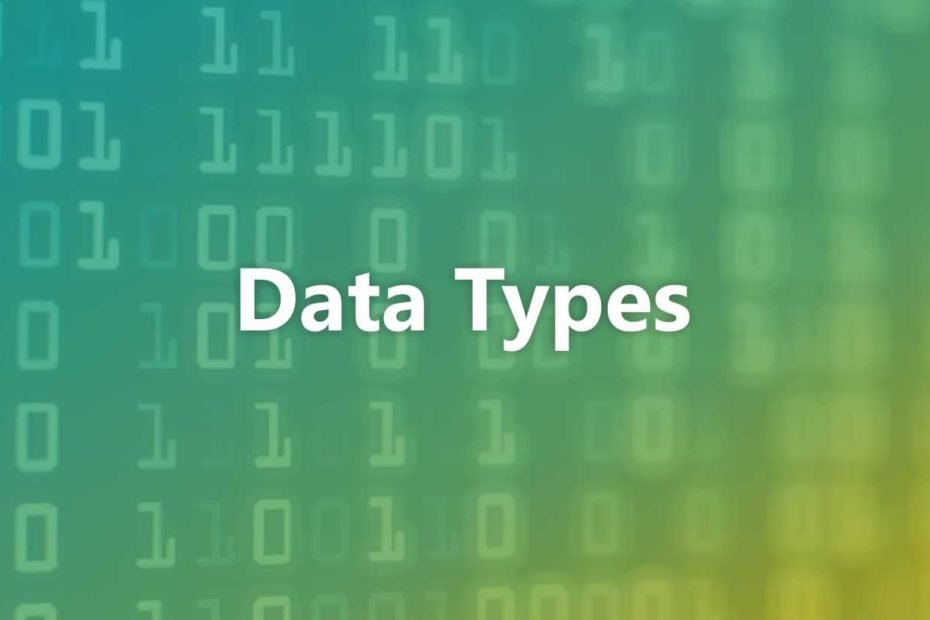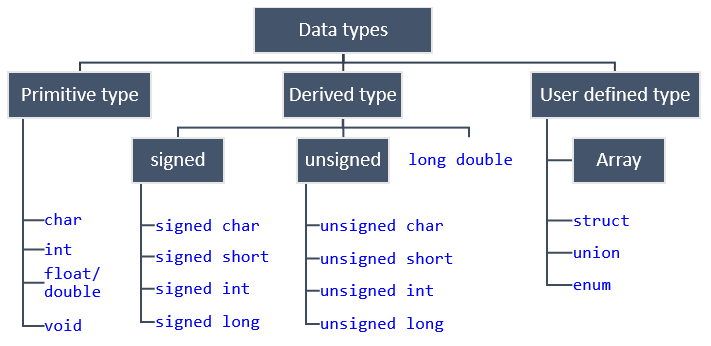# Data Types Programmers Must KnowAre you planning to become a programmer? If so, you need to learn data types first.

Why is that? Because the data type is a classification that determines how you will use the data in the program .

So, by using the right data type, the program you create will run smoothly.

Well, actually there are so many programming data types themselves. But in this article we will only discuss the most common types, namely types that fall into the primitive and composite categories .

Within these two categories, there are six commonly used data types, namely:

1. Integer ( integer )
2. Fractional numbers ( floating point )
3. Karakter ( Char )
4. Boolean
5. String
6. Array

Have you ever heard of the six data types? Want to know more? Let’s see in the next section!

## 6 Data Types Programmers Need to Learn

We will now discuss the details of each type. First, we will discuss four data types that fall into the primitive category, namely integer, floating point, char, and boolean .

Finally, we will discuss two types that fall into the composite category, namely strings and arrays .

Here are the differences between primitive data and composite data:

• Primitive – This type is generally a built-in of a programming language .
• Composite – This type is a combination of primitive data. This type of combination is also known as a data structure.

OK, without further ado, let’s get started!

### 1. Integer ( Integer )

Integers are used to display numbers without decimal places . Examples such as 123, 85723, or 5. You can also use it for numbers that are minus as long as the number does not contain decimals, such as -19 or -2362.

Well, there are four types of integers, namely byte, short, int, and long . Here are the sizes and ranges of each type:

As you can see, the lower the data size and the higher the range. Size is the volume of data, while range is the range of values.

For example, suppose the data value is 200, then the data cannot fit into the byte type . Because the byte range is only from -128 to 127 only.

### 2. Fractions ( Floating Point )

Next there are fractional numbers, aka floating point . As the name implies, this type displays numbers that contain decimals . Examples are 3.14, 7.5, or -5.6.

Unlike integers, floating point only has two types, namely float and double . They can be distinguished by their size, range, and number of digits:example data type in C

### 3. Character ( Char )

Unlike integers and floating point , char is a data type that allows you to store character information.

The types of characters also vary. Ranging from letters and numbers to special characters such as punctuation marks.

In addition, this type also has no variations:

### 4. Boolean

The last data primitive is a boolean . This type is the simplest form. Because its function is only to declare whether a value is true ( true / 1) or false ( false / 0).

### 5. String

Okay, now we go to composite data. The first composite data type we will cover is string . Because string is one of the most popular types.

If a char can only represent one character, a string can be used to store a set of characters.

Here are the differences in the values ​​of char and string :

• Char: A, B, C, 1, 2 dan 3
• String: “Hello World” or “Test 123”

### 6. Array

An array is a data type that contains a set of variables of the same type. So, if you see a set of data that is of a different type, it’s not an array .

For example, suppose you store the values ​​”C”, “D”, “E”, “F” and “G” in a variable of type array . That is, the set of values ​​can also be called an array of characters . This is because the values ​​”C” to “G” are values ​​of the same type, namely char .

## Use the Right Data Types when Building Programs!

Now you know which data type is right for a certain value or variable? So, don’t use the wrong type, because this can affect the performance of the program you make.

In addition to types, you also need to learn different types of programming languages . Of course, the type of programming language you learn must also be in accordance with your career path .